# FinishedProbability for IVs on PRO

#### Kaminokage

##### Veteran Trainer
SUMMARY

• I. Introduction
• II. What are IVs?
• III. Method 1 : TOTAL 5 IVs
• IV. Probability for TOTAL 1 to 6 IVs
• V. Method 2 : IV Probability
• VI. ReferencesI. Introduction

As we can notice, it’s very hard a find an epic PKM: Have the right ability, have the right nature, and have epic IVs. The concept of epic depends of each person, we don’t have the same perception of what PKM is epic or not. We have an idea of the probability to find one PKM with the rarity tiers (it’s not precise sadly), we know the probability for ability (H.A. = 5%), we all use Synchronize to find the right nature with 50% probability, but nobody did a guide about the probability for IVs. So, I decided to post two methods to apprehend the rarity of different PKM based on IVs. It’s not really a guide, there is no method to have better IVs on PKM, and my post will not give the answer to the big question: “Is my PKM epic?”. All my guide is only one indicator of rarity.II. What are IVs?

IVs means Individual Value, you can find it on your PKM in orange :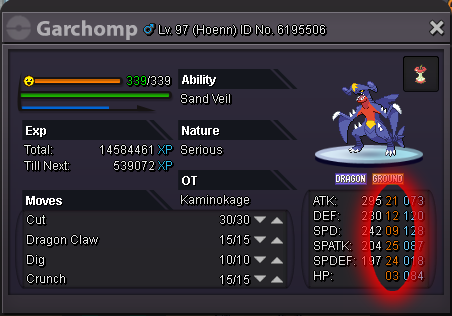IVs are predefined values from 1 to 31, they are random generated when you engage a PKM in the wild. You can’t change it, a PKM born and die with his IVs.
With a value from 1 to 31 in the 6 different statistics, there are 31^6 = 887 503 681 combinations of IVs. It means every PKM is almost unique for his IVs.
Other interesting fact: the combination 31 in each stats has a probability of 1 / 887 503 681. And the sum of the ID PKM for Red, Blue and Yellow Server is around 50m, so we are not even close to find one perfect PKM in IVs :devil:

What is the point to have “good” IVs?

The all point to have “good” IVs is to have better stats for fight. You can see the formula to calculate the statistic of a PKM :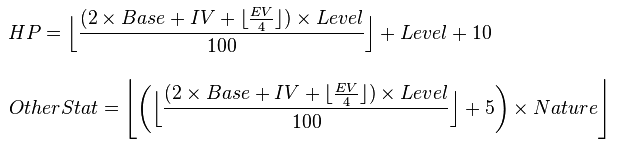I will take an example with the statistic ATK for a PKM lv 100 with 80 Base Stat (average of Base Stat), 252 EV and Adamant nature: [/B]
• ATK = ( ( ( 2 * base + IV + EV/4 )* Lv ) / 100 + 5 ) * Nature
• ATK = ( ( ( 2 * 80 + IV + 252/4 ) * 100 ) / 100 + 5 ) * 1,1
• ATK = ( ( (223 + IV ) + 5 ) * 1,1
• ATK = 250,8 + IV *1,1

So, in lv 100, the IVs will add 1 stat for each IV. And for a bonus nature, it will add 1,1 stat for each IV.
If my PKM, in the example, has 26 in IVs ATK, he will have 250,8 + 28,6 = 279,4 ATK.

To resume, having goods IVs in each stats can help in fight, but the influence is not huge. So, you don’t need the perfect IVs on your PKM, you just need to have correct IVs.III. Method 1: TOTAL 5 IVs.

I will base this part of the guide on the majority of PKM : for a lot of PKM, one statistic is useless.
An example: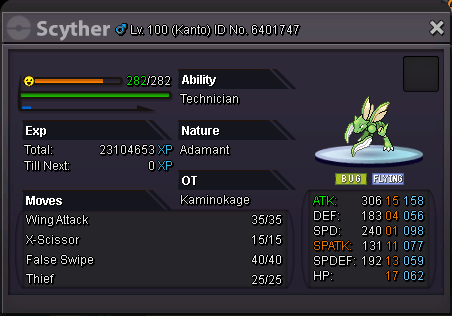For Scyther, the statistic SPATK is useless, he can have 1 or 31 IVs, this statistic will never be use. So, the TOTAL 5 IVs is the sum of his IVs except the useless statistic.
In my example, for Scyther : TOTAL 5 IVs = 50 (ATK 15 + DEF 04 + SPD 01 + SPDEF 13 + HP 17)
The same method can be use with another TOTAL IVs, depends of the PKM you select.

Imagine, I don’t like this Scyther, his ability and nature are perfect, but his IVs seems bad for me. I don’t care about having 31 in ATK and SPD, I just want have a TOTAL 5 IVs = 120 at least. But, before I chase one with this TOTAL 5 IVs, I want to estimate how much time I will take to chase it. So, I need to calculate the probability to have at least a TOTAL 5 IVs = 120.

How can I calculate it?

The IVs are randomly generate like dices, it’s as if I have 5 dices with 31 sides and I want the number of time I can have the sum 120 for this dices.
The probability is determinate by this formula: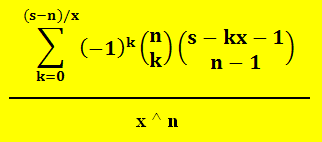With :
• s = sum wanted => 120
• n = number of dices => 5
• x = number of sides => 31
• k = all value undivided 0 to (s – n) / x

So, I need to do the sum of probability TOTAL 5 IVs = 120 to 155, and I will have my probability to have a TOTAL 5 IVs = 120 at least.

Lucky me, I have Excel. And the answer to the sum of probability TOTAL 5 IVs = 120 to 155 is 2,296%.
So, if I want a Scyther with a sum of 120 in his 5 useful stats, I have 2,296% to have it. In average, I need to catch 44 Scyther Technician Adamant before I can have one with TOTAL 5 IVs = 120.

What is the point to know this?

The TOTAL 5 IVs is one indicator of rarity, it will no help to chase better IVs, you can be lucky and find it in first try or catch 500 before have it. But, it’s a tool for decision-making: Should I buy or chase? The TOTAL 5 IVs gives you a view of how much time you will take for chase a PKM, and help you determinate the price you can pay for buying a PKM.

It can be also a tool for chasing a PKM: for a PKM rarity tiers 1, it’s easy to catch a lot, so for a first selective sorting of your catches, you can decide what percentage of PKM you will release before looking in specifics IVs.
For example : I will keep only the 10% better PKM in TOTAL 5 IVs, the 10% matches to TOTAL 5 IVs = 107. So, I will release all PKM under this sum and only after I will look in specifics IVs. Because even if a Scyther has 31 in ATK and SPD, with TOTAL 5 IVs = 107, he will have only 45 in the rest of his stats.

The TOTAL 5 IVs has a big disadvantage, this method doesn’t take into account specifics stats. Two PKM can have the same TOTAL 5 IVs, but one can have 31 in a vital statistic when the other PKM will have 1. It’s up to you to use your brain after using the method x)IV. Probability for TOTAL 1 to 6 IVs

In the previous part, we saw what is the TOTAL 5 IVs, in this part, i publish the probabilities associates to each TOTAL IVs.
It's easy to use, you look for the TOTAL IVs you want and go look to the sum to know what is his "rarity". For example: TOTAL 5 IVs = 90 => 32,222%, it means that 32,222% of PKM has same or better TOTAL 5 IVs than him.

The red lines delimit the percentages: 1% / 2% / 3% / 5% / 10% / 20% / 30%.

TOTAL 1 IVs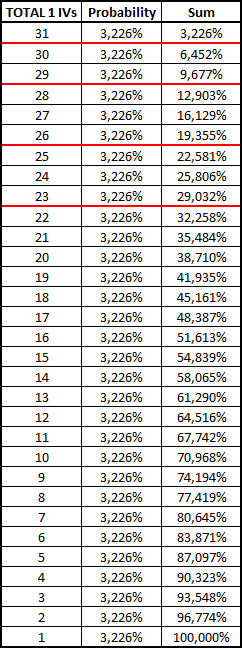TOTAL 2 IVs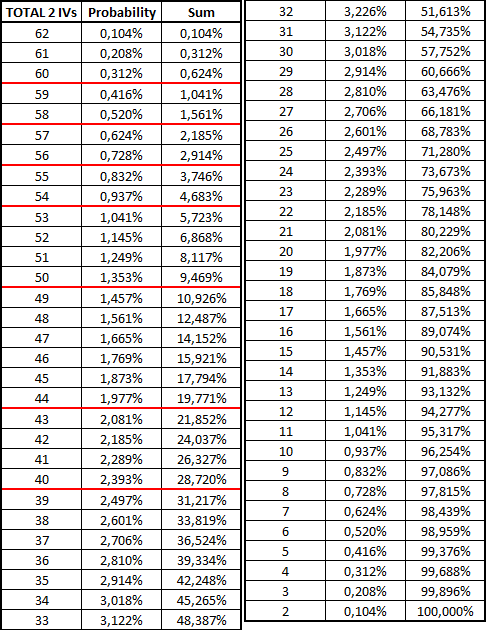TOTAL 3 IVs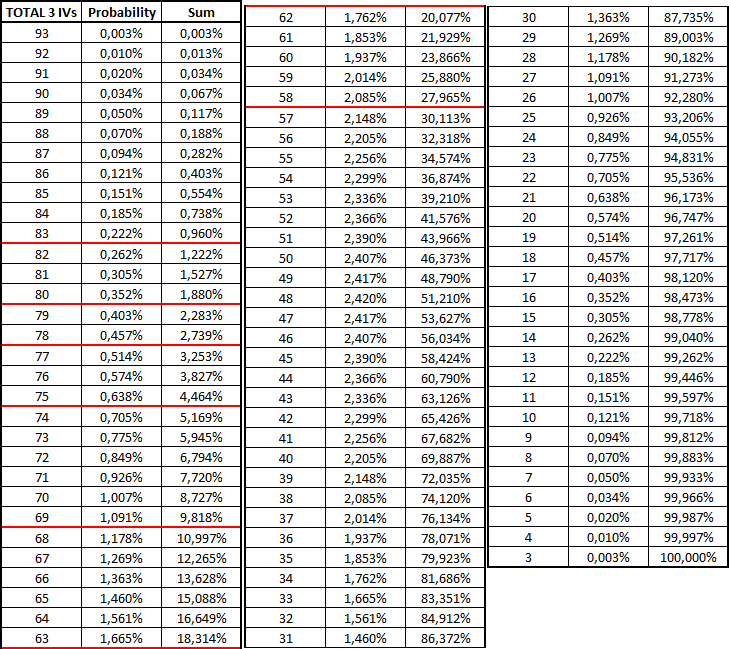TOTAL 4 IVs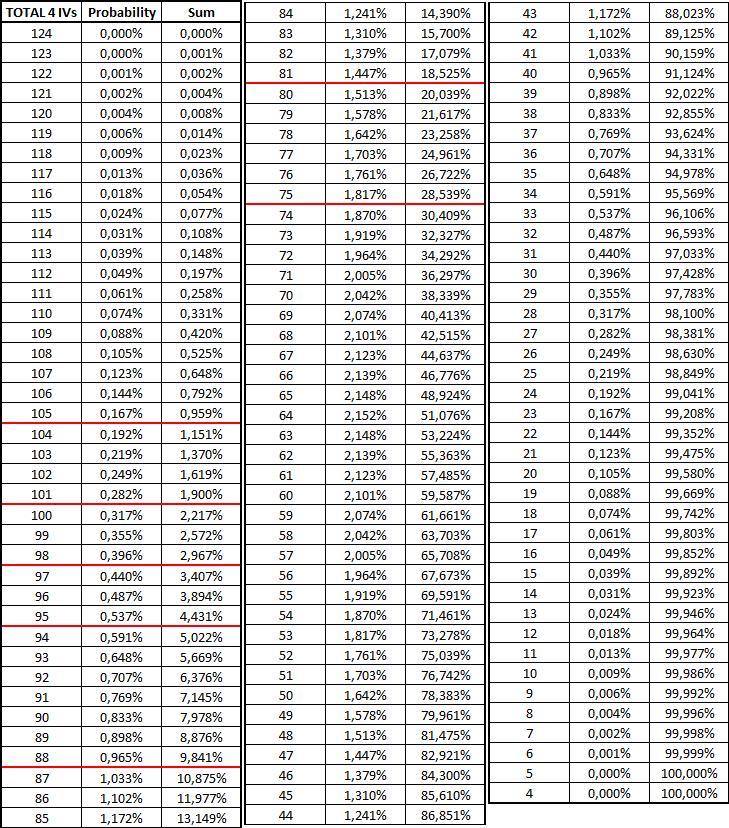TOTAL 5 IVs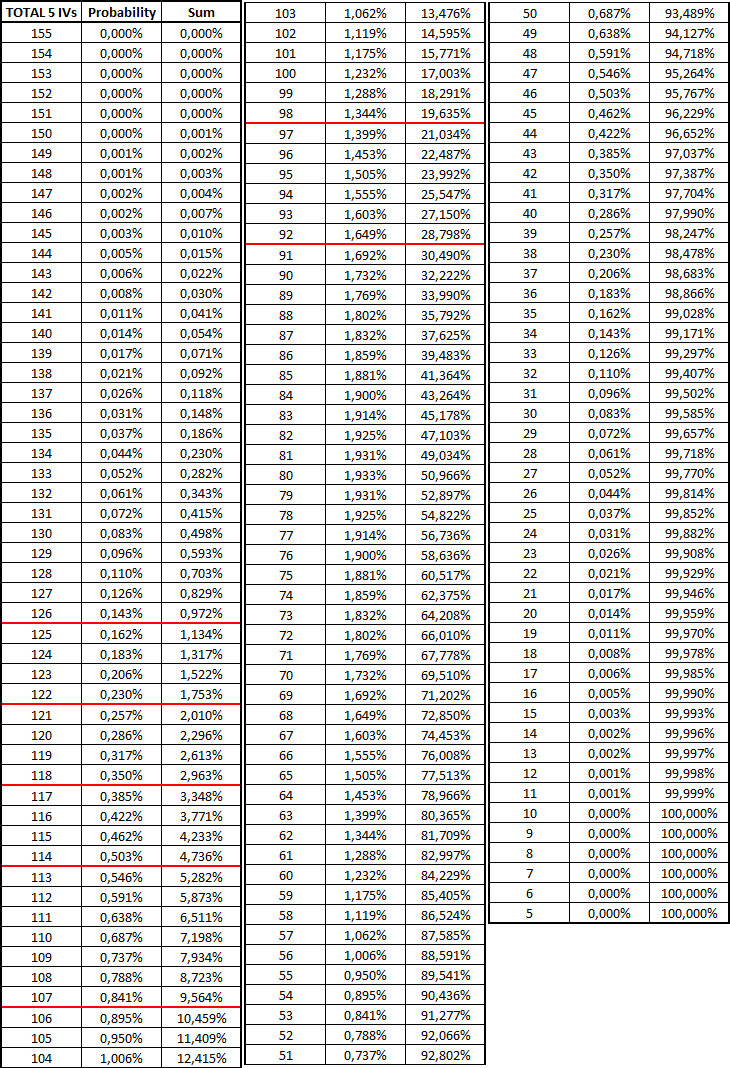TOTAL 6 IVs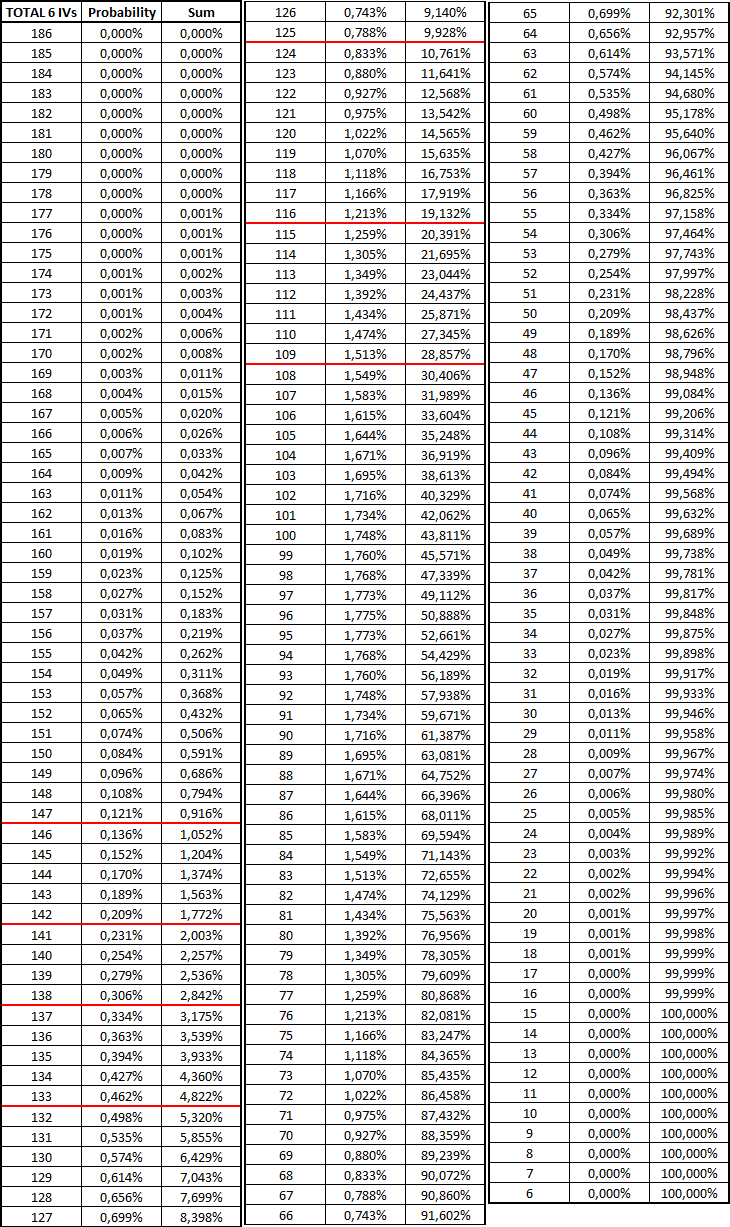I share the Excel i used, so you can use it too.
https://www.dropbox.com/s/e4dvjolkk5p1b26/PROBABILITY IVS.xlsx?dl=0
In the right top, you can click on download. If something is wrong with the Excel or the uploader, tell me.

Or you can use the online calculator i made :
T5IVs Calculator by Kaminokage : http://fsogame.free.fr/tools/calct5ivs.phpV. Method 2 : IV Probability

As we saw previously, the TOTAL 5 IVs doesn’t take into account specifics stats, so another method exist to evaluate one PKM. You can calculate directly the probability for specifics IVs.
The formula is :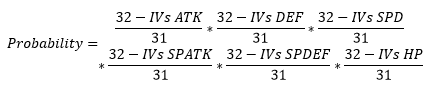This formula is good if you want to use the 6 IVs to calculate your probability, but if you want only 5 IVs (for example: Scyther again), you can remove the part with the useless stats (SPATK for Scyther). This easy remove is explain because you don’t care about your SPATK, you can have 1 or 31 it’s the same: if IVs SPATK = 1, so (32-1)/31 = 1, and multiply by 1 changes nothing to the probability x)

I will take an example of using this method:

Same than before, I want a Scyther, but this time I want in IVs:
• At least 30 in ATK
• At least 20 in DEF
• At least 30 in SPD
• At least 20 in SPDEF
• At least 20 in HP

I calculate his probability:
• IVs Proba = ((32-ATK)/31)* ((32-DEF)/31)* ((32-SPD)/31)* ((32-SPDEF)/31)* ((32-HP)/31)
• IVs Proba = ((32-30)/31)* ((32-20)/31)* ((32-30)/31)* ((32-20)/31)* ((32-20)/31)
• IVs Proba = (2/31)* (12/31)* (2/31)* (12/31)* (12/31)
• IVs Proba = 0,000241432 = 0,0241%

So, it means than 0,0241% of Scyther will have exactly or better than the IVs I want. So I need to farm in average 4142 Scyther to have it.

This method seems nice, but it has disadvantage too. You can’t use it for evaluate the value of your PKM. This method is too much selective. I will take another example to explain it:My dear Scyther, again, if I calculate his IVs Proba with this method I obtain: 14,6894%
This result seems absurd, it would mean 85,32% of Scyther are worse than him. It’s because, with this method, only better IVs than 17 in HP are selected. A lot of part of Scyther are deleted without taking care of the rest of his IVs.

It’s the same for a PKM with 31/1/1/1/1/1 in IVs, the method will only select the PKM with 31 IVs in ATK, so the result will be 3,2258%.
Even if the value seems nice, the PKM is trash. So, again, it’s up to you to use your brain after using the method x)

You can find in my Excel a Calculator for this IVs Proba.VI. References

Formula for statistics : https://bulbapedia.bulbagarden.net/wiki/Statistic
Link to my Excel : https://www.dropbox.com/s/e4dvjolkk5p1b26/PROBABILITY IVS.xlsx?dl=0
T5IVs Calculator by Kaminokage : http://fsogame.free.fr/tools/calct5ivs.phpThanks you for reading, if you see some errors or want to add an other method (or something else) to this post, don't hesitate to contact me :)
And sorry for my english, it's not my native language :(

Last edited:
•Sylveon and Mutantnair

#### PreHax

##### Legendary Trainer
Donator
Re: Probability for IVs in PRO

<r><QUOTE author="Kaminokage" post_id="376335" time="1492674520" user_id="1488380"><s>
Kaminokage post_id=376335 time=1492674520 user_id=1488380 said:
</s><br/>
.<br/>
<e>
</e></QUOTE><br/>
<br/>
Again Kaminokage; such a good guide <E>:Heart:</E></r>

#### Sameen

##### Newbie Trainer
I cant understand it fully.

#### Kaminokage

##### Veteran Trainer
Neel123456 post_id=484836 time=1514053395 user_id=1566913 said:
I cant understand it fully.
It was the easy way :Frown:
But i will try to resume it.

I created two methods to determinate the probability to have one PKM with some IVs. The main constraint being that each combination of IVs is almost unique, each combination has a probability of 1/887 503 681, from there, it"s impossible to deduce anything from it. But on a global reasoning, it becomes easier to calculate probabilities.

The method 1: TOTAL 5 IVs will take only the sum of IVs on the 5 useful statistics, on my example, i took a Scyther Adamant, the useless statistic will be the SPATK, so i do the sum of his 5 others statistics. When i have this sum, i can calculate the probability associated with this sum :It's the column "sum"; example, my sum of TOTAL 5 IVs is 120 (to have at least 120 in total 5 IVs), the probability will be: 2,296%.

It means, in average, if you want a specific PKM with 120 (at least) in sum on his 5 useful statistic, you will need to catch 44 PKM to have 1. Of course, it's only probabilities, you can have 3-4 PKM with 120 T5IVs on your 44 PKM.

I particularly like this method because it's very close to the "reality" of farm. When we farm a lot of PKM, this method is very close to the result of your farm. But like i already say: it stays only one indicator of rarity.

If someone finds a method much closer to reality, i will be happy to read it :y:

Thanks a lot

#### Kaminokage

##### Veteran Trainer
Update formatting with new forum.

#### Kaminokage

##### Veteran Trainer
My old uploader for pictures was close, so all pictures were deleted. Hopefully, i still had this pictures.

Update :

#### AleksanderRoman

##### Newbie Trainer
Donator
This is truly a masterpiece, well written for players with some knowledge.
Thank you sir.

#### Extremtarz

##### Youngster
Amazing information great work...

#### Kaminokage

##### Veteran Trainer
Once again, my picture uploader closed, so i reuploaded all pictures, hope it's the last time, pray for Gyazo to stay alive for a long time xD

Update :